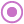#If (PQL)

Control the flows of logic by executing certain statements if the criteria expression is true, and other statements if it is false.

• Returned Output: Variant
• Library: PQL \ Semantic \ Logical
• Version: 2018.00.000
• Compatibility: Pyramid Query Language (PQL) data sources
• Click here to review the functional operators covering arithmetic, comparison, and logical operations.
• Logical operators used for composite logical and comparative expressions: && = 'AND';  || = 'OR'

#### Syntax

If( <Criteria> , <TRUE> , <FALSE> )

* Click on the function's arguments above for more details on the input values.

• The logical criteria expression is a condition that evaluates to true or false.
• If the logical expression is true, the true expression is evaluated and returned.
• If the logical expression is false, the false expression is evaluated and returned.
• The true or false expressions can evaluate to numeric values (including data points and tuples), or to list/ set definitions for producing a list of model elements from a hierarchy.
• If the expression results are list or set definitions, the final resolved element list must come from the same model hierarchy.
• The true and false expressions should return the same type of result: either numeric values (and data points) or sets. Mixing result types can lead to errors.
• For details on how to employ and use this function see the semantic calculation overview.
• Click here to review the functional operators covering arithmetic, comparison, and logical operations.
• Logical operators used for composite logical and comparative expressions: && = 'AND';  || = 'OR'
##### Different Function types
• This function is similar the MDX 'iif' function.
• The common library offers an identical function for non-model based date-time strings. See this function.
• The SWITCH function is also similar to 'if'.

#### Building Logical Expressions

Logical expressions are usually comparative expressions that evaluate to a 'true' or a 'false'. Comparison operators like ">", "<" and "=" are usually required to build such expressions. Multiple comparative expressions can be blended together using Logical operators like "&&" (AND) and "||" (OR) to build sophisticated expressions.

#### Examples

This example evaluates the cost of blade products. If it's over 500,000, then the formula will return the value of Australian Sales plus 5. Otherwise, it will return the value of Canadian Sales:

If( ([Product].[Product].[Blade], [measures].[cost]) > 500000, ([Customer].[Country].[Australia], [measures].[sales]) + 5, ([Customer].[Country].[Canada], [measures].[sales]) )

This example evaluates 2 conditions: the cost of blade products and overall sales. If blade costs are less than or equal to 100,000 AND ("&&") sales are greater than 20,000, then the formula will return a list of the top 3 manufacturers based on August 2018 expenses. Otherwise, it will return a list of all manufacturers:

If( ([Product].[Product].[Blade], [measures].[cost]) <= 100000 && [measures].[sales] > 20000, TopCount({AllMembers([manufacturers].[manufacturer])},3,([measures].[data Expenses], [data].[dateKey].[aug 2018])), {AllMembers([manufacturers].[manufacturer])} )

This example evaluates 2 conditions: overall sales and overall quantity. If sales are greater than 20,000 OR ("||") quantity is equal to 500, then the formula will return Canadian sales. Otherwise, it will evaluate another nested "If" method.

The nested function will check if overall expenses are NOT EQUAL ("!=") to 1500. If that is true, it will return UK Sales. Otherwise, it will return 25000 as a flat value:

If( [measures].[sales] > 20000 || [measures].[quantity] = 500, ([Customer].[Country].[Canada], [measures].[sales]), If( [measures].[expenses] != 1500, ([Customer].[Country].[UK], [measures].[sales]), 25000 ) )